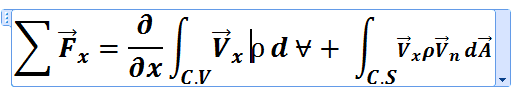# Integral form of momentum equation

Ali Durrani
Hello guys!
can i get the derivation for this equation ?## Answers and Replies

Mentor
I think the first term should be a partial derivative with respect to time t (not x), and represents the rate of accumulation of x-momentum within the control volume. The second term represents the net rate of x-momentum leaving the control volume.

•Delta2
Ali Durrani
I think the first term should be a partial derivative with respect to time t (not x), and represents the rate of accumulation of x-momentum within the control volume. The second term represents the net rate of x-momentum leaving the control volume.
yes sir as you said the 1st term should be the partial derivative with respect to time but i don't know why our teacher in the fluid class wrote the equation in this form, and it was not a mistake because he used it again and again, can you explain the logic behind it as he is a very senior teacher and he wouldn't make such a silly mistake

Mentor
yes sir as you said the 1st term should be the partial derivative with respect to time but i don't know why our teacher in the fluid class wrote the equation in this form, and it was not a mistake because he used it again and again, can you explain the logic behind it as he is a very senior teacher and he wouldn't make such a silly mistake
I stand by what I said. I can't account for what your teacher does. All I can do is confidence in what I am saying.

My questions for you are:

1. How does his equation account for the time rate of accumulation of x momentum within the control volume?

2. When he writes the corresponding equation for the conservation of mass within the control volume, does his equation have a partial derivative with respect to x or a partial derivative with respect to t?

Mentor
Here's a couple of more questions for you:

1. What are the units of the term as your teacher has written it?

2. Are those units of force?

Mentor
So.... What are your answers to my questions?

Ali Durrani
sorry i asked him and he said its partial by partial t and not x his writing style is different so i mistakenly wrote it as partial by partial x

•Chestermiller
Mentor
sorry i asked him and he said its partial by partial t and not x his writing style is different so i mistakenly wrote it as partial by partial x
No problem.